﻿﻿ Inductance of Nonlinear Material (B-H Curve)examples｜products｜Murata Software Co., Ltd.# Example19Inductance of Nonlinear Material (B-H Curve)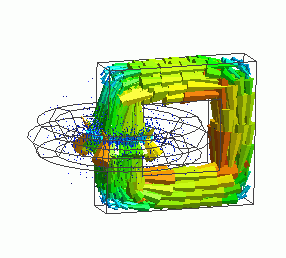General

• Inductance of a nonlinear material (permeability is defined with B-H curve) is solved.

• The vectors of the magnetic field and the magnetic flux density are solved.

• Unless specified in the list below, the default conditions will be applied.

### Analysis Space

 Item Settings Analysis Space 3D Model unit mm

### Analysis Conditions

 Item Settings Solvers Magnetic Field Analysis [Gauss] Analysis Type Static analysis Options N/A

### Model

A loop coil (coil) and a magnetic core (core) with gap are placed.

As the magnetic field concentrates in the gap of the magnetic core, small mesh size is applied (0.2mm).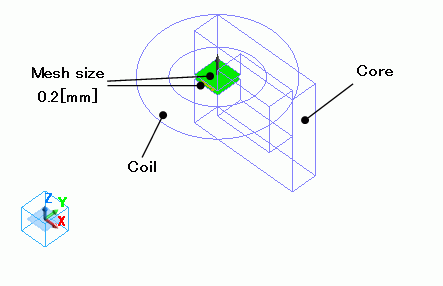### Body Attributes and Materials

 Body Number/Type Body Attribute Name Material Name 9/Solid Coil 008_Cu * 8/Solid Core Core

* Available from the Material DB

Material property of the core is defined with B-H curve table.

• If the magnetization characteristic is defined by B-H curve or M-H curve, calculation is nonlinear. Nonlinear calculation may not converge.
In such case, refer to “If the Nonlinear Calculation (B-H Curve) Does Not Converge “

Material Name

Tab

Settings

Core

Permeability

Magnetization Characteristic Type: Select B-H curve

B-H Curve Table

 Magnetic Field [A/m] Magnetic Flux Density [T] 0 0 58 0.42 90 0.8 180 1.19 380 1.37 1100 1.48 2000 1.55 3000 1.608 11000 1.81 20000 1.91 80000 2.15

Press the Graph button. The following graph will show up.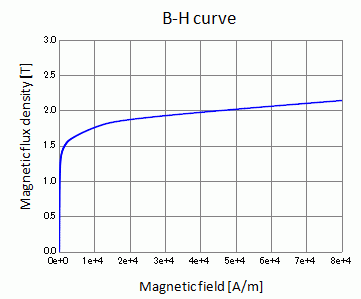Body attribute is set up as follows to apply current to the loop coil.

 Body Attribute Name Tab Settings Coil Current Waveform: Constant Current: 1[A] Turns: 1[Turns] Direction: Loop Coil/Magnetic Field Direction Magnetic Field Vector: X=0, Y=0, Z=1

No setting.

### Results

To see the results of inductance calculation, go to the [Results] taband click [Table]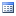.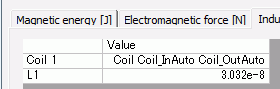The vectors of the magnetic flux density are shown below.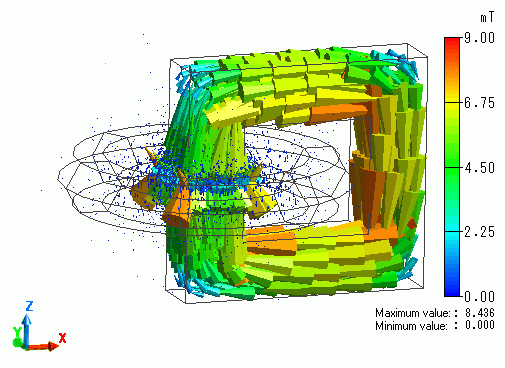The section at XZ plane.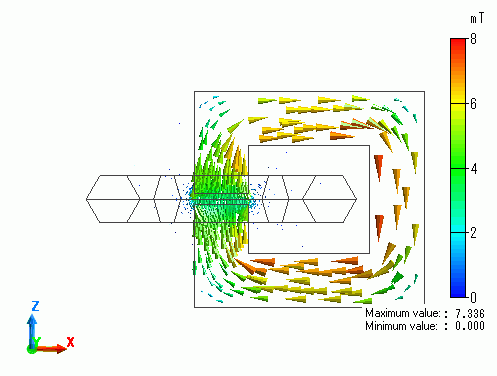﻿ ﻿